# Dielectric Material as an Electric Field Medium

Dielectric materials are materials having no free electrons to conduct. The main difference from insulators is that it can store energy. All the insulators in electrical circuits are dielectrics. The change of dielectric material with and without electric field is represented in figure 1.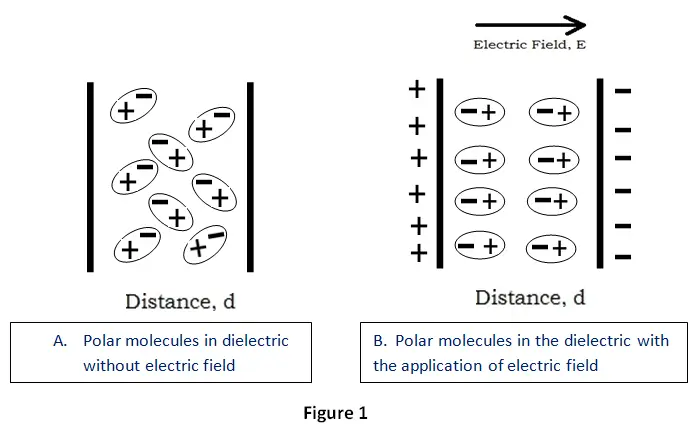In the steady operating condition, the role of the dielectric material is as an electric field medium. This material is actually placed between two current carrying metal plates. The plates together with the material form a capacitor.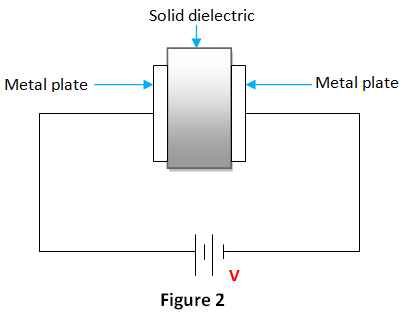Now, consider two metal plates. An ideal solid dielectric is placed between the plates. The material having zero electrical conductivity is taken. Then, an alternating voltage is given across the plates.
As a result, an alternating field is developed in the solid dielectric. Thus a wattles capacitance current will flow in the dielectric material. It is reactive in nature. The capacitive current will lead the voltage by 90o. It is given by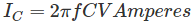C → Capacitance of capacitor (F)
f → Frequency (Hertz)
V → Voltage applied (V)

This current will regularly flow till the application of voltage. This is not the case when DC voltage is applied. This capacitance current will flow only till the development of electric field in the solid dielectric and the charging of capacitor will be full. That is, as time increases charging of capacitor increases. The charge is given by
Q = CV (at the end of charging period)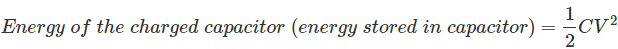This energy is reversible. That is; when it gets discharged, the energy stored will regress.
Now, we consider a dielectric with electric conductivity is not zero. That is, a practical dielectric is considered. Then, there will be another active current other than the wattles capacitive current. It is given by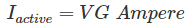G → Admittance (inverse of impedance)
When a voltage is applied across a dielectric at normal condition; that is the dielectric used in the capacitor is of good operating state, then the energy taken from the supply consist of reversible energy and non reversible energy. The former is necessary to develop the electric field in dielectric and the latter is to cover the losses in dielectric. The energy losses are through dispersal and the energy converted to heat. The losses are called as dielectric losses. This is high in high frequency.

Now, the capacitance of a capacitor with and without a dielectric material is considered below. In the first case; with the air as medium and it is given by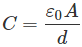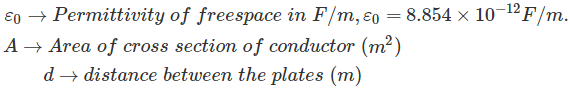In the second case, a capacitor with a solid dielectric material is considered. Here, the capacitance get increased by a factor, k. The capacitance is given by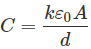The factor is called dielectric constant. It is a constant which gives the characteristics of the dielectric material.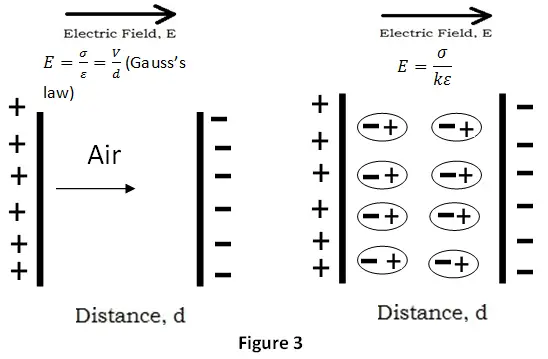Here, we can see the effects of dielectric material as an electric field medium. The effects are;

1. The electric field (E) will decrease with the addition of dielectric material, when the charge is kept invariable.
2. The capacitance, C will increase with the use of dielectric material.

Want To Learn Faster? 🎓
Get electrical articles delivered to your inbox every week.
No credit card required—it’s 100% free.